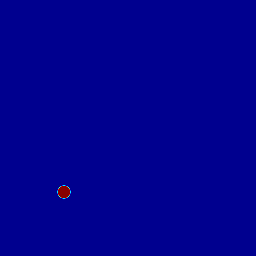# Accelerating droplet

A drop moves at constant velocity u.x = 0.5, u.y = 0.5 on a fixed grid. The density ratio is 1000. With the non-momentum conserving formulation a droplet is formed and accelerates, which is clearly not physical.Non-momentum conserving formulation

This looks much better when using the momentum-conserving formulation.Momentum conserving formulation

#define MOMENTUM 0

#if MOMENTUM
#  include "momentum.h"
#else
#  include "navier-stokes/centered.h"
#  include "two-phase.h"
#endif

#define Re 2000.
#define vrho1 (1.)
#define vrho2 (1./1e3)

#define ycc 1.
#define xcc 1.

int main (int argc, char * argv[])
{
init_grid (256);
size (4.);

rho1 = vrho1, rho2 = vrho2;
mu1 = vmu1, mu2 = vmu2;

run();
}

event init (t = 0) {
fraction (f, sq(radius) - sq(x - xcc) - sq(y - ycc));
#if MOMENTUM
foreach() {
rhov[] = rho(f[]);
q.x[] = rhov[]*f[]/2.;
q.y[] = rhov[]*f[]/2.;
}
boundary ({f,q,rhov});
#else
foreach() {
u.x[] = f[]/2.;
u.y[] = f[]/2.;
}
boundary ({f,u});
#endif
}

event snapshot (t = 0.; t += 0.03; t <= 2.35) {
static FILE * fp = popen ("ppm2gif > f.gif", "w");
output_ppm (f, fp, min = 0, max = 1);
}